## The Heisenberg Uncertainty Principle

The wave packets we tried above satisfy an uncertainty principle which is a property of waves. That is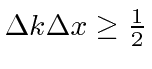.

For the square'' packet the full width in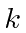is. The width inis a little hard to define, but, lets use the first node in the probability found at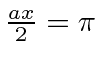or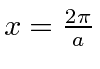. So the width is twice this or. This gives uswhich certainly satisfies the limit above. Note that if we change the width of, the width of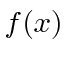changes to keep the uncertainty product constant.

For the Gaussian wave packet, we can rigorously read the RMS width of the probability distribution as was done at the end of the section on the Fourier Transform of a Gaussian.We can again see that as we vary the width in k-space, the width in x-space varies to keep the product constant.The Gaussian wave packet gives the minimum uncertainty. We will prove this later.

If we translate into momentum, thenSo the Heisenberg Uncertainty Principle states.It says we cannot know the position of a particle and its momentum at the same time and tells us the limit of how well we can know them.

If we try to localize a particle to a very small region of space, its momentum becomes uncertain. If we try to make a particle with a definite momentum, its probability distribution spreads out over space.

Jim Branson 2013-04-22